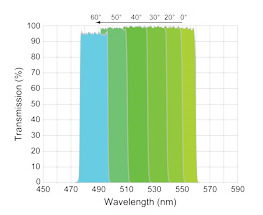Switch Customer
• ##### {{category.Description}}View All Resources

# Filter Spectra at Non-normal Angles of Incidence

While most applications call for optical filters to be used at normal incidence, it is important to understand how the spectral properties of different types of filters change when using these filters a non-normal angles of incidence (AOI).  There are two main effects exhibited by all filter spectrum as the angle is increased from normal:

1. The features of the spectrum shift to shorter wavelengths.

2. Two distinct spectra emerge – one for s-polarized light and one for p-polarized light.

## RazorEdge® Edge Filters

The graph on the right (Figure 1) shows a series of spectra derived from a typical RazorEdge long-wave-pass (LWP) filter design, and can be applied to any of the RazorEdge edge filters.  Here, the resulting wavelength λ is compared to the wavelength λ0 of the edge location at normal incidence. As the angle increases from normal incidence, the filter edge shifts toward shorter wavelengths and the edges associated with s- and p-polarized light shift by different amounts. For LWP filters, the response of p-polarized light shifts more than s-polarized light. The opposite is true for SWP filters. This polarization splitting causes the unpolarized spectrum to show a "shelf" near the 50% transmission point, but note that the edge steepness remains intact, even for polarized light.

The shift of almost any spectral feature can be approximated by a simple model of the wavelength of the feature vs. angle of incidence, given by the equation: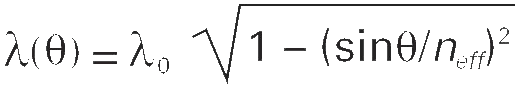where neff is the effective index of refraction, which varies with AOI and polarization, and λ0 is the wavelength of the spectral feature of interest at normal incidence.  For the RazorEdge family of  edge filters, the shift of the 90% transmission point on the edge is described by this equation with neff = 2.08 and 1.62 for s- and p-polarized light, respectively. To learn more about determining the spectral change of RazorEdge filters, click here.

## MaxLine® Laser-line Filters

Varying filter designs will respond to changes in AOI in different ways, but still show marked differences between s- and p-polarized light.  The spectra of a MaxLine laser-line filter (Figure 2) shows that as the angle increases from normal incidence, the center wavelength shifts toward shorter wavelengths and the bandwidth broadens slightly for p-polarized light while narrowing for s-polarized light. The most striking feature is the decrease in transmission for s-polarized light, while the p-polarized light maintains high transmission.  The center wavelength shifts are described by the above equation with neff = 2.19 and 2.13 for s- and p-polarized light, respectively, for many of the MaxLine filters.  To learn more about how the spectral features of the MaxLine filters will change with AOI, please contact our Applications Engineers.

## StopLine® Notch Filters

The StopLine series of filters has a different response to AOI depending on the grade of filter used.  For the standard U- and S-grade filters (Figure 3), as the angle is increased from normal incidence, the notch center wavelength shifts to shorter wavelengths, the notch depth decreases, and the notch bandwidth decreases (with a greater decrease for p-polarized light than for s-polarized light). The shift of the notch center wavelength is described by the above equation with neff = 1.76 for both s- and p-polarized light.

E-grade filter (Figure 4), which is based on a different type of filter design, shows a large increase in OD for s-polarized light as the AOI increases. As the angle is increased from normal incidence, the notch center wavelength shifts to shorter wavelengths; however, the shift is greater for p-polarized light than it is for s-polarized light. The shift is described by the above equation with neff = 1.71 for p-polarized light and neff = 1.86 for s-polarized light.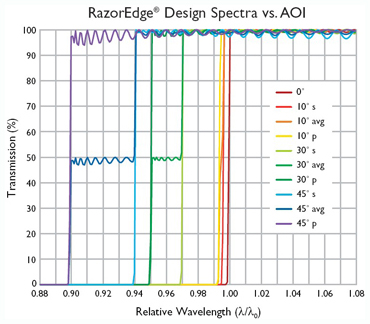Figure 1: Two distinct filter spectra emerge for s- and p-polarized light as AOI increases.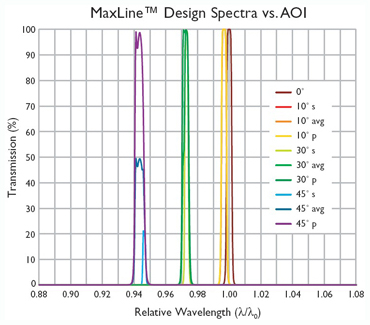Figure 2: MaxLine filter spectral response to AOI shift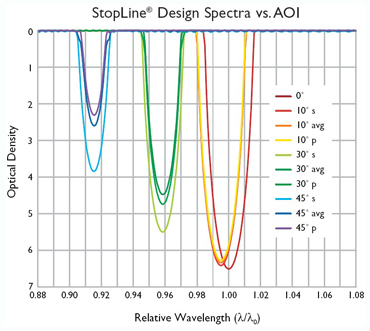Figure 3:  U- and S-grade StopLine filter spectra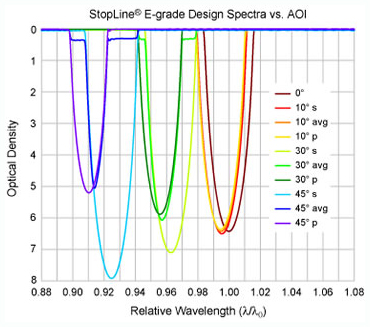Figure 4:  E-grade StopLine filter spectra

## Modeling Data for non-normal angles of incidence

Try angle tuning on selected filters, by using MyLight™. Select your filter and click on the blue "MyLight" button at the top right of the filter spectra to model the theory data at whatever angle you desire.

## VersaChrome® Tunable Filters

Generally speaking, the spectral response to changes in AOI is dependent upon the type of filter design.  While standard bandpass filters will exhibit distortion and polarization splitting as the AOI increases, the VersaChrome tunable filters are designed to maintain high transmission, steep edges and polarization insensitivity from 0° to 60°, allowing the user to angle tune the filter according to his needs.